Publication date: 03/23/2021

##Statistical Details for Estimation Methods

Penalized regression methods introduce bias to the regression coefficients by penalizing them.

###Ridge Regression

An l2 penalty is applied to the regression coefficients during ridge regression. Ridge regression coefficient estimates are given by the following: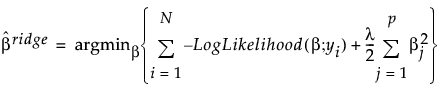,

where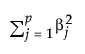is the l2 penalty, λ is the tuning parameter, N is the number of rows, and p is the number of variables.

###Dantzig Selector

An l penalty is applied to the regression coefficients during Dantzig Selector. Coefficient estimates for the Dantzig Selector satisfy the following criterion: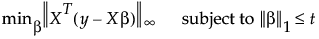where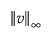denotes the l norm, which is the maximum absolute value of the components of the vector v.

###Lasso Regression

An l1 penalty is applied to the regression coefficients during Lasso. Coefficient estimates for the Lasso are given by the following: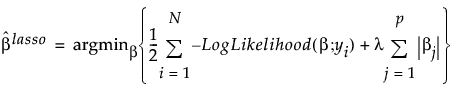,

where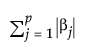is the l1 penalty, λ is the tuning parameter, N is the number of rows, and p is the number of variables

###Elastic Net

The Elastic Net combines both l1 and l2 penalties. Coefficient estimates for the Elastic Net are given by the following: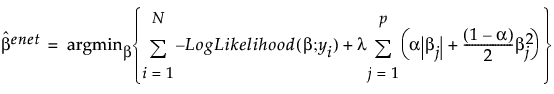,

This is the notation used in the equation:is the l1 penaltyis the l2 penalty

λ is the tuning parameter

α is a parameter that determines the mix of the l1 and l2 penalties

N is the number of rows

p is the number of variables

Tip: There are two sample scripts that illustrate the shrinkage effect of varying α and λ in the Elastic Net for a single predictor. Select Help > Sample Data, click Open the Sample Scripts Directory, and select demoElasticNetAlphaLambda.jsl or demoElasticNetAlphaLambda2.jsl. Each script contains a description of how to use it and what it illustrates.

###Adaptive Methods

The adaptive Lasso method uses weighted penalties to provide consistent estimates of coefficients. The weighted form of the l1 penalty is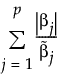,

where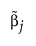is the MLE when the MLE exists. If the MLE does not exist and the response distribution is normal, estimation is done using least squares andis the solution obtained using a generalized inverse. If the response distribution is not normal,is the ridge solution.

For the adaptive Lasso, this weighted form of the l1 penalty is used in determining the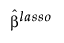coefficients.

The adaptive Elastic Net uses this weighted form of the l1 penalty and also imposes a weighted form of the l2 penalty. The weighted form of the l2 penalty for the adaptive Elastic Net is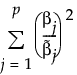,

whereis the MLE when the MLE exists. If the MLE does not exist and the response distribution is normal, estimation is done using least squares andis the solution obtained using a generalized inverse. If the response distribution is not normal,is the ridge solution.

Want more information? Have questions? Get answers in the JMP User Community (community.jmp.com).